val：一个表示 Node.val 的整数。 random_index：随机指针指向的节点索引（范围从 0 到 n-1）；如果不指向任何节点，则为 null 。 你的代码 只 接受原链表的头节点 head 作为传入参数。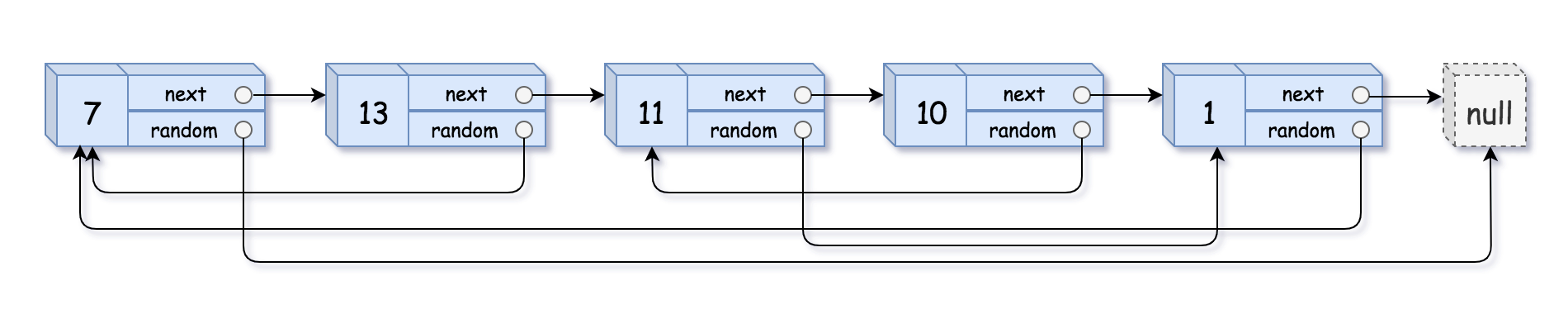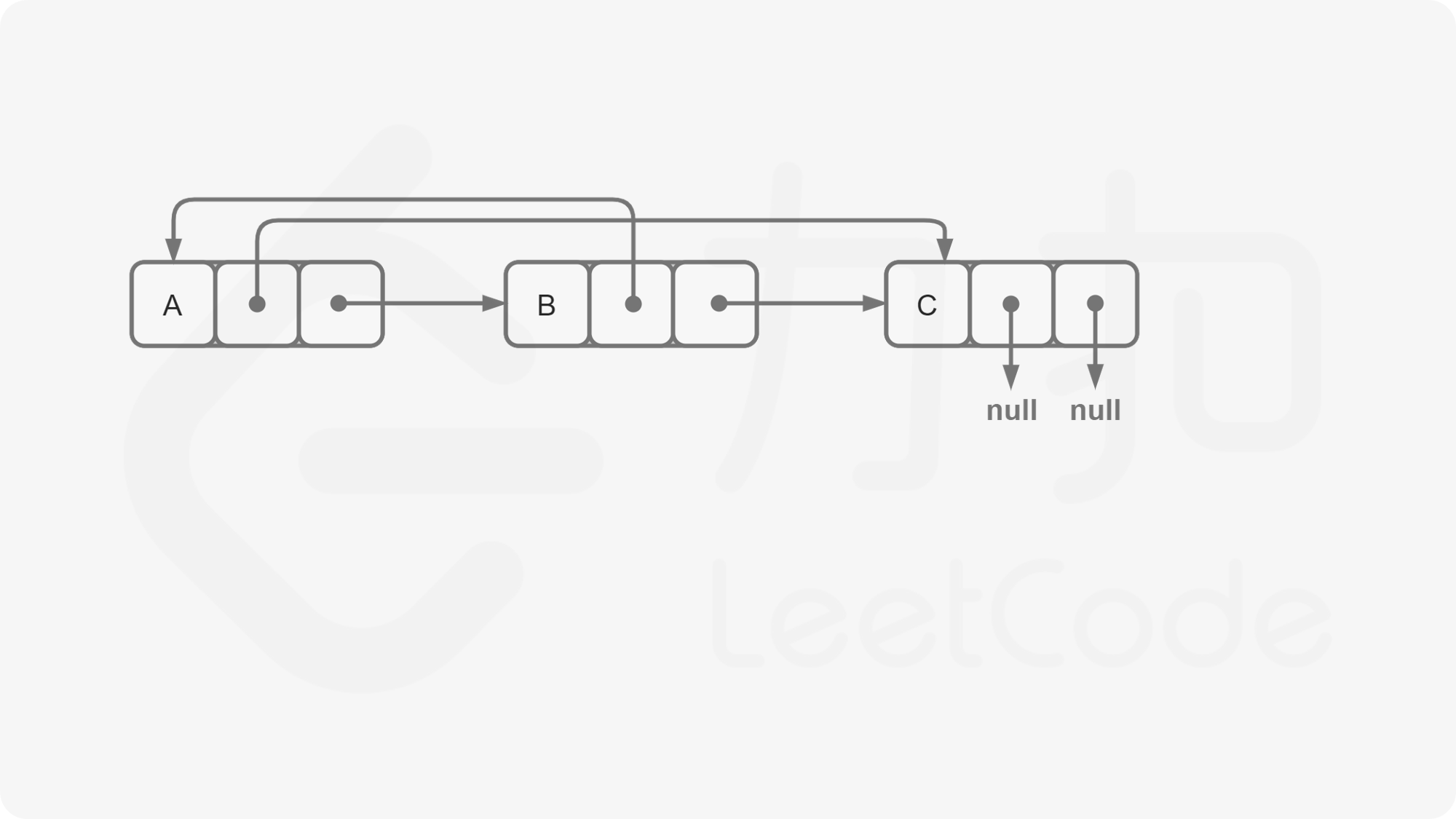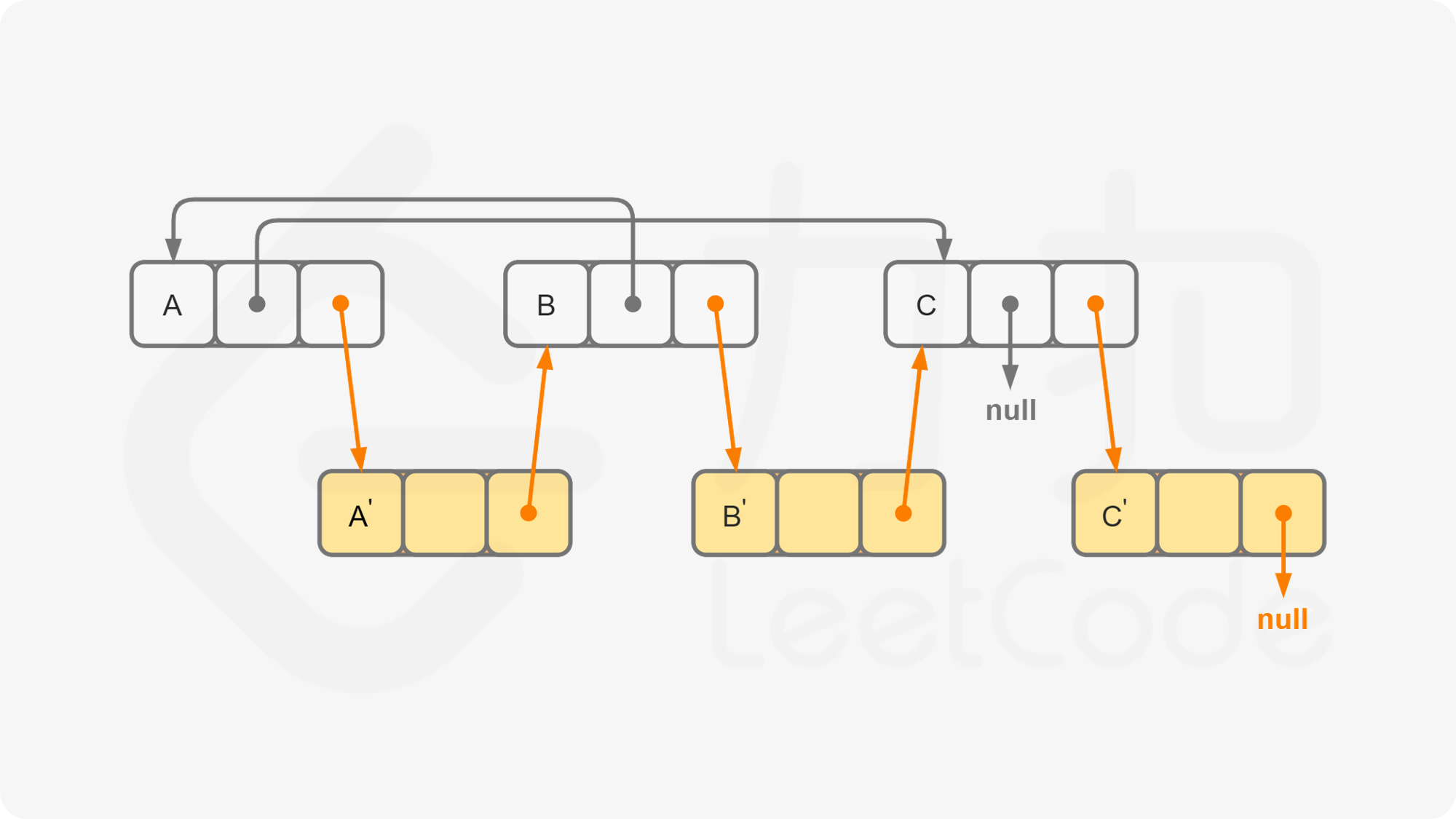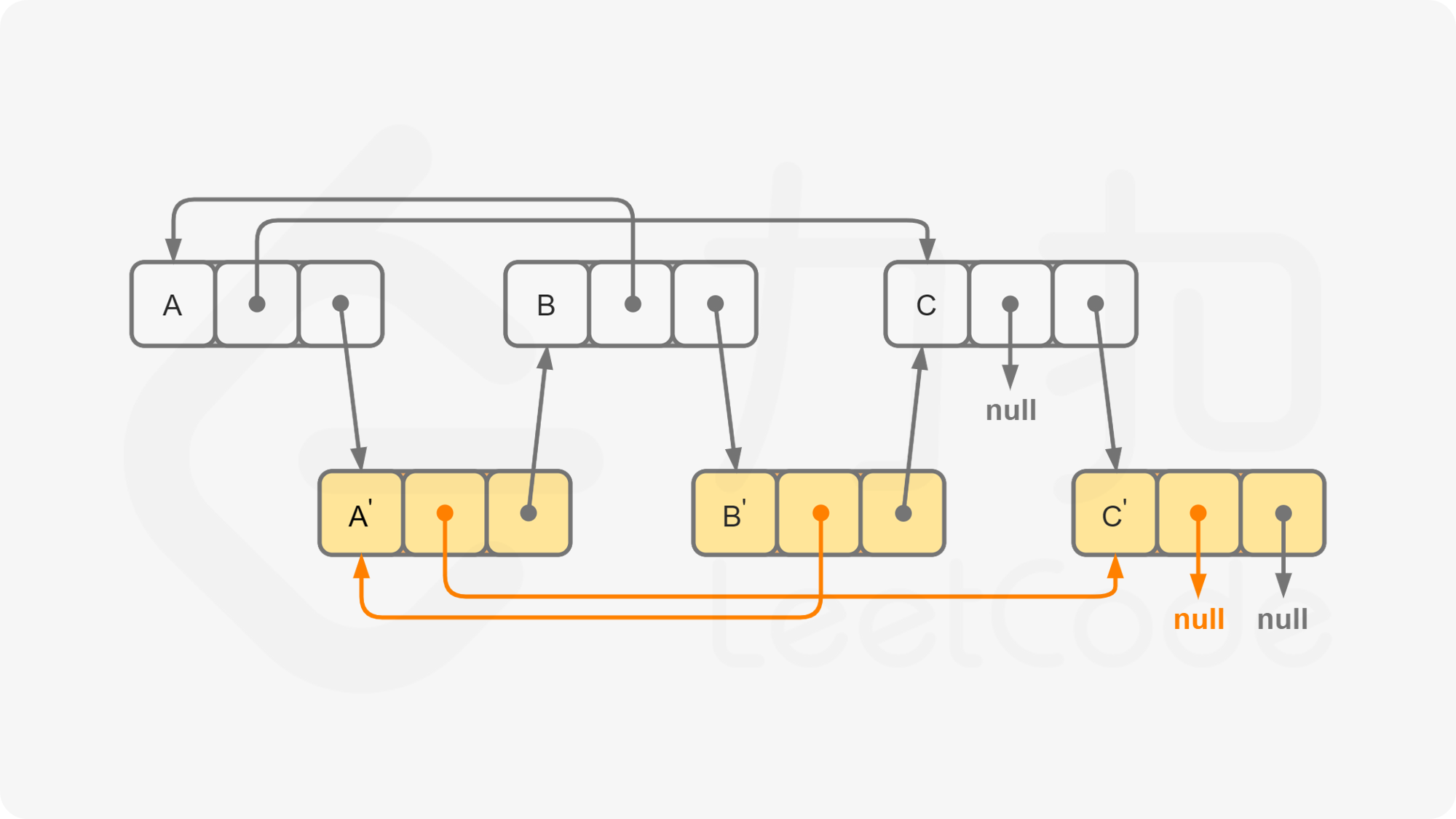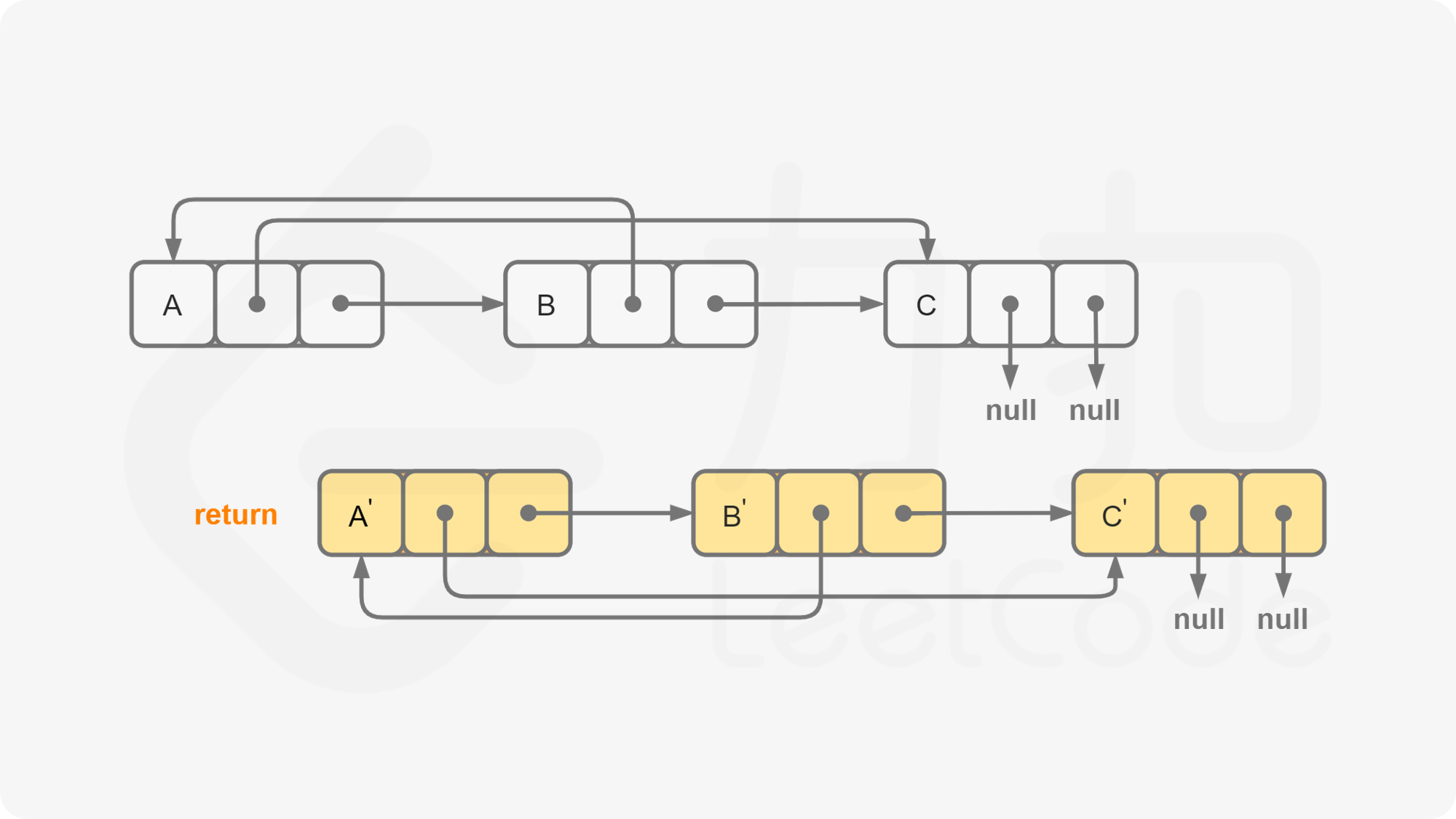class Node:
def __init__(self, x: int, next: 'Node' = None, random: 'Node' = None):
self.val = int(x)
self.next = next
self.random = random

class Solution:
def copyRandomList(self, head: 'Node') -> 'Node':
return None
while p:
new_node = Node(p.val,None,None)
new_node.next = p.next
p.next = new_node
p = new_node.next
while p:
if p.random:
p.next.random = p.random.next
p = p.next.next

return dummy.next